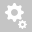##Recently viewed tickets1. Loads considered in Construction Stage and Post CS are different
2. Consideration of Locked in stresses

1. Loads considered in Construction Stage and Post CS are different

All the static loads (like element, line, nodal, etc. ) when activated in construction stage are by default clubbed under, CS: Dead Load. Which implies, then results due to all these static loads, self weight of structure, crash barrier load, wearing course load, etc. would be displayed under CS: Dead Load case of last Construction Stage and not just the self weight.

On the contrary, in Post CS stage, the static load cases (say, ST: Dead Load) would represent a user defined static load case, which might just include the self weight and hence the difference.

output’ box in the Construction Stage Analysis Control dialogue box as shown below.

Go to Analysis>‘Construction Stage Analysis Control’2. Consideration of locked in stresses

Construction stage analysis would also incorporate the time dependent effects of creep, shrinkage as well as compressive strength. The dead load calculations for elements activated under construction stage, are based on the age of the concrete on the day when the element is activated . However, in Post CS, the strength of concrete taken for the particular element is that at the end of last construction stage.
Let us consider the simply supported beam loaded with 20kN/m. The maximum deflection for the same could be calculated and compared, based on the age at which the beam is
loaded. Elastic Modulus of concrete is directly proportional to its compressive strength.The equation to calculate maximum deflection of simply supported beam with UDL is :Also the maximum deflection is inversely proportional to Elastic Modulus of concrete. Hence, later the age of loading, greater is the Elastic Modulus, and, lesser the deflection.

Now say, this beam has 2 construction stages of 3 days and 1000 days. So, defining the static Dead Load case under the type ‘Construction Stage Load (CS)’ and activating it when age of concrete is 3 days, Elastic Modulus of concrete at 3 days (E3) would be considered for deflection calculation. However, checking the same in Post CS, Elastic Modulus corresponding to the age, at the end of 2nd stage, i.e., 1003 is considered.To eliminate such issues, it is recommended, the loads which are to be activated under construction stage be defined as Type
‘Construction Stage